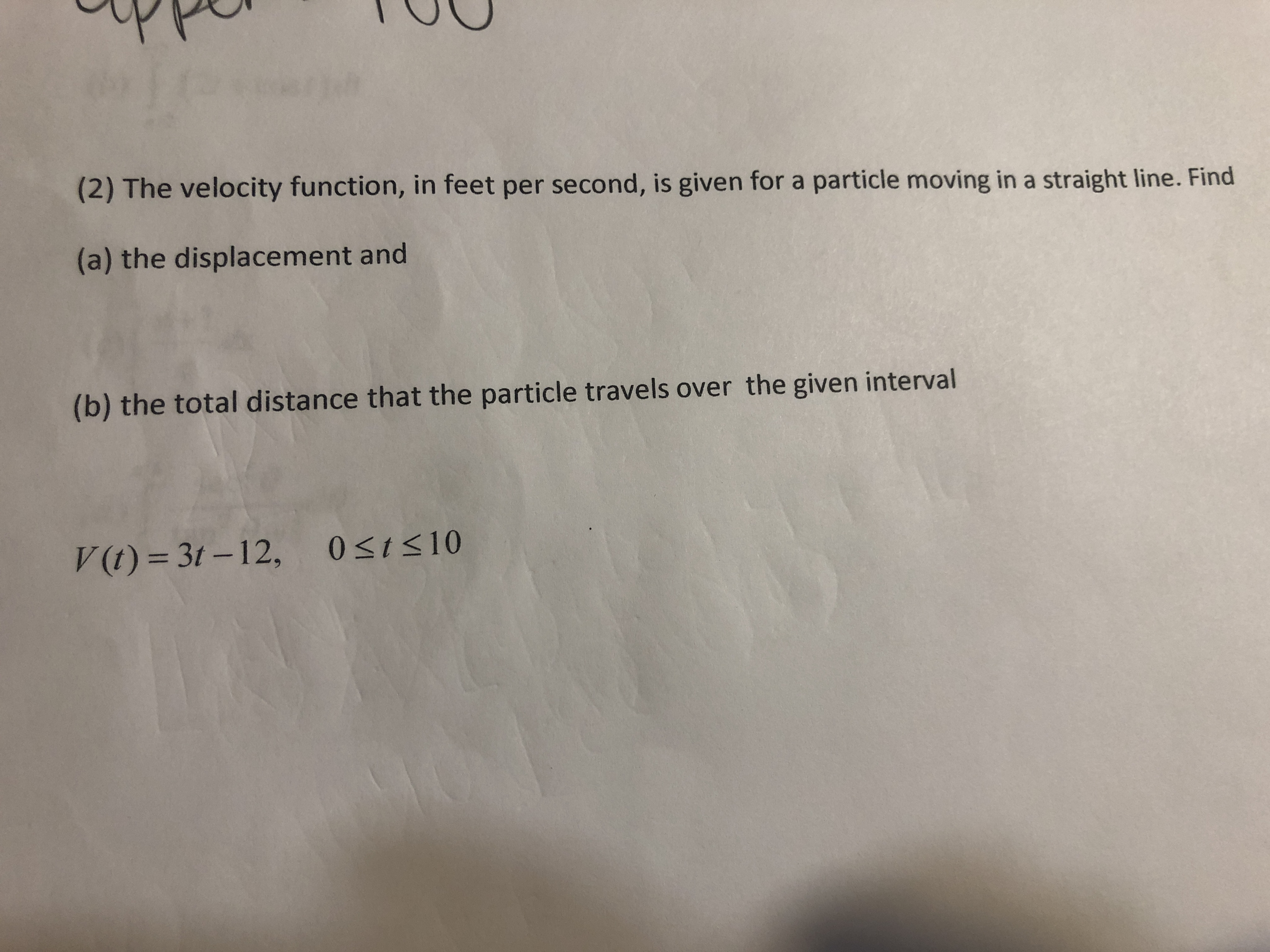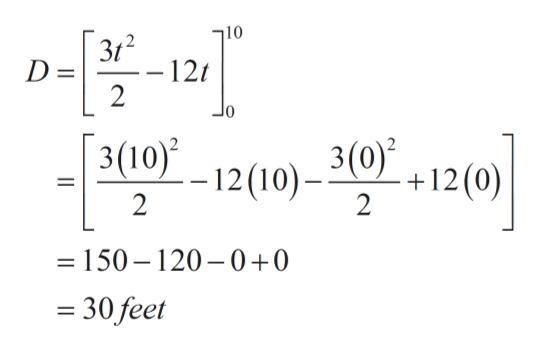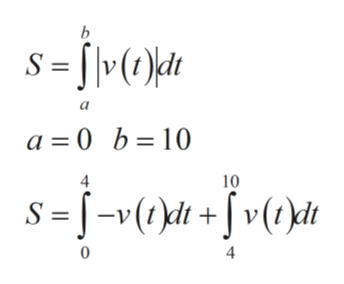# (2) The velocity function, in feet per second, is given for a particle moving in a straight line. Find(a) the displacement and(b) the total distance that the particle travels over the given intervalV (t) = 3t – 12,0st

Question
22 viewshelp_outlineImage Transcriptionclose(2) The velocity function, in feet per second, is given for a particle moving in a straight line. Find (a) the displacement and (b) the total distance that the particle travels over the given interval V (t) = 3t – 12, 0st<10 fullscreen
check_circle

Step 1

Displacement ‘D’ of a particle moving from point ‘a’ to point ‘b’ in a straight line with velocity ‘v’ is given by the formula. Using these values given to find displacement ‘D.

Step 2

Solving for displacement ‘D’ using basic definite integration.help_outlineImage Transcriptionclose312 D = – 12t 10 3(10) 3(0) - 12(10)– 2 +12(0) 2 = 150 – 120–0+0 = 30 feet || fullscreen
Step 3

Distance ‘S’ travelled by a particle form point ‘a’ to point ‘b’ in a straight ...help_outlineImage Transcriptionclose8 = j•O\« v(1 )dt a = 0 b=10 4 10 -v(t)dt+ S = 4 fullscreen

### Want to see the full answer?

See Solution

#### Want to see this answer and more?

Solutions are written by subject experts who are available 24/7. Questions are typically answered within 1 hour.*

See Solution
*Response times may vary by subject and question.
Tagged in

### Integration